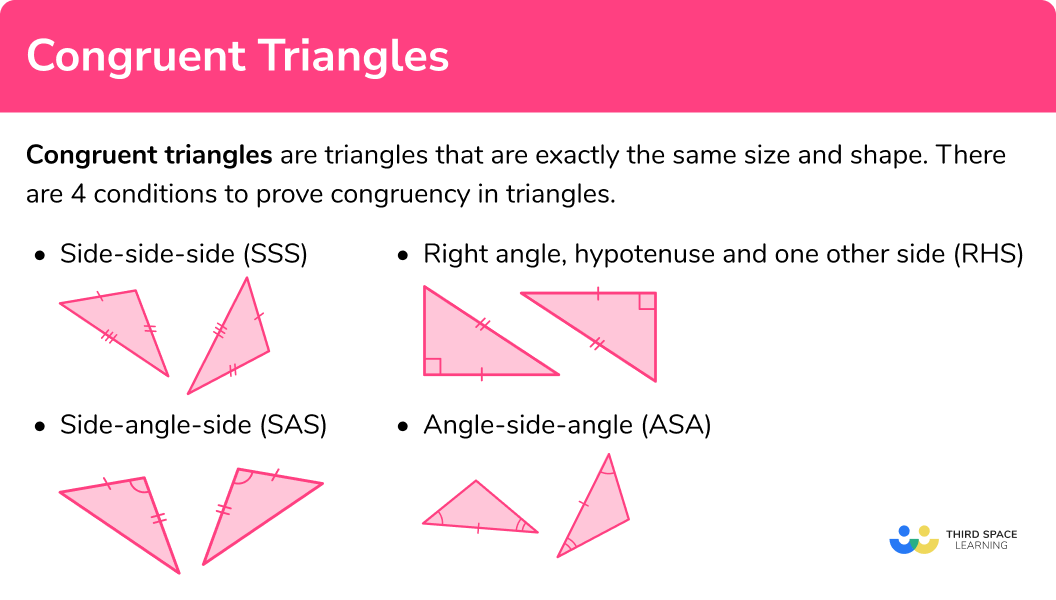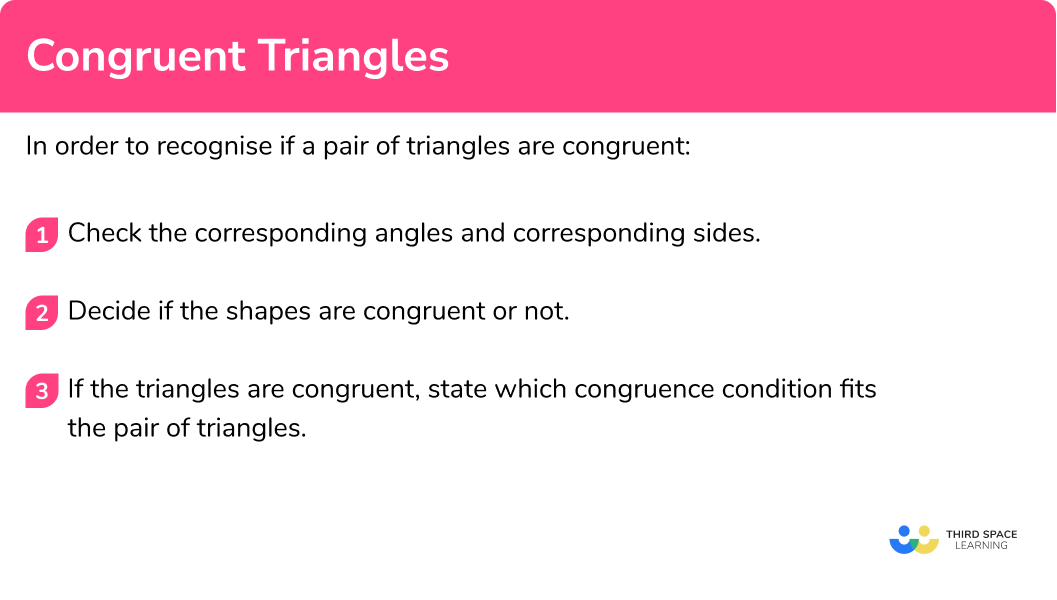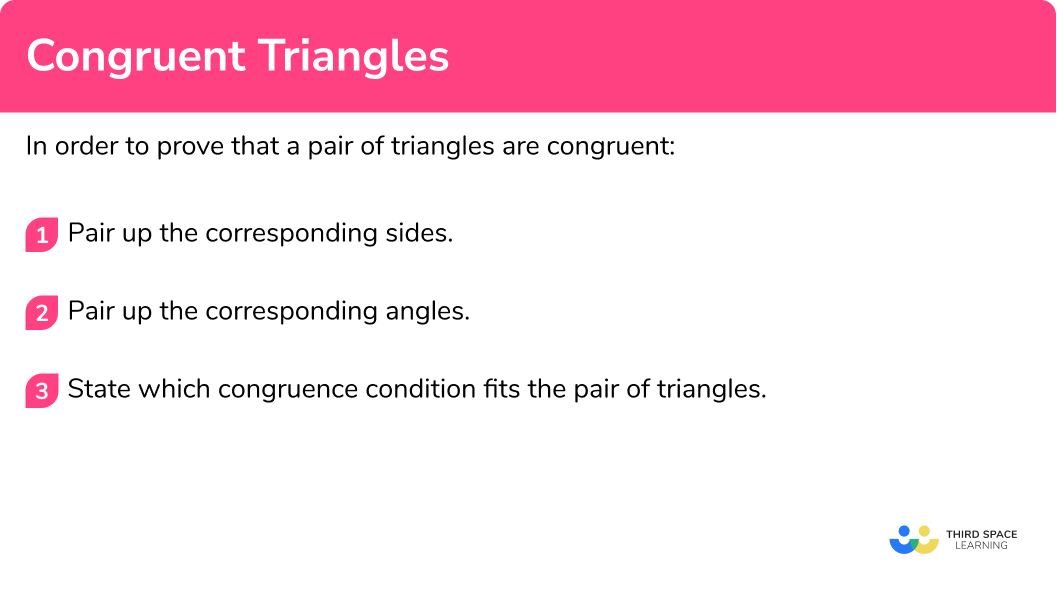# Congruent Triangles

Here we will learn about congruent triangles, including how to identify them and prove the congruence of triangles.

There are also congruent triangles worksheets based on Edexcel, AQA and OCR exam questions, along with further guidance on where to go next if you’re still stuck.

## What are congruent triangles?

Congruent triangles are triangles that are exactly the same size and shape.

There are 4 conditions to prove congruency in triangles.

### Side-side-side (SSS)

When two triangles have all three sides the same, they are congruent triangles.

The second triangle may be a rotation or a mirror image of the first triangle (or both).

### Right angle, hypotenuse and one other side (RHS)

When two triangles are right-angled triangles and have the hypotenuse and one of the shorter sides the same, they are congruent triangles.

The second triangle may be a rotation or a mirror image of the first triangle (or both). The third side would also be identical and this can be checked using Pythagoras’ theorem.

### Side-angle-side (SAS)

When two triangles have two sides and the included angle the same, they are congruent triangles. The included angle is the angle in between the two sides.

The second triangle may be a rotation or a mirror image of the first triangle (or both).

### Angle-side-angle (ASA)

When two triangles have two angles and the included side the same, they are congruent triangles. The included side is the side in between the two angles.

The second triangle may be a rotation or a mirror image of the first triangle (or both).

This can also be known as angle-angle-side (AAS) as if two angles in a triangle are known, the third angle can be worked out using the angle fact that the sum of interior angles in a triangle is 180° .

### What are congruent triangles?### Keywords

Congruent is used to describe shapes such as quadrilaterals or polygons which are exactly the same shape and the same size.

Similar is used to describe shapes such as quadrilaterals or polygons which are the same shape, but different sizes.  A scale factor is involved.

## How to recognise congruent triangles

In order to recognise if a pair of triangles are congruent:

1. Check the corresponding angles and corresponding sides.
2. Decide if the shapes are congruent or not.
3. If the triangles are congruent, state which congruence condition fits the pair of triangles.

### How to recognise congruent triangles### Related lessons on congruence and similarity

Congruent triangles is part of our series of lessons to support revision on congruence and similarity. You may find it helpful to start with the main congruence and similarity lesson for a summary of what to expect, or use the step by step guides below for further detail on individual topics. Other lessons in this series include:

## Congruent triangles examples

### Example 1: recognise congruent triangles

Decide whether this pair of triangles are congruent. If they are congruent, state why:

1. Check the corresponding angles and corresponding sides.

Both triangles have sides 5cm and 7cm .  They both have an angle of 95° .

2Decide if the shapes are congruent or not.

The 95° angles are in a corresponding position. The triangles are mirror images of each other. The triangles are congruent.

3If the triangles are congruent, which congruence condition fits the pair of triangles.

The triangles are congruent with the condition side-angle-side (SAS).

### Example 2: recognise congruent triangles

Decide whether this pair of triangles are congruent. If they are congruent, state why:

Both triangles have sides 6.3cm, 8.1cm and 10.2cm .

The triangles are the same shape and the same size – they are congruent.

The triangles are congruent with the condition side-side-side (SSS).

### Example 3: recognise congruent triangles

Decide whether this pair of triangles are congruent. If they are congruent, state why:

Both triangles have sides 8cm .

Both triangles have a 50° angle.

But their second angles are different.

The triangles look like they are different shapes BUT the third angle can be worked out.

Using the angle fact that the sum of interior angles of a triangle is 180° we can work out the missing angle in both triangles.

The 8cm side is in between the 30° and 50° angles in both triangles. The triangles are the same shape and the same size.

They are congruent triangles.

The triangles are congruent with the condition angle-side-angle (ASA).

### Example 4: recognise congruent triangles

Decide whether this pair of triangles are congruent. If they are congruent, state why:

Both triangles are right-angled triangles.

They both have a short side of 9cm .

But the hypotenuse of each triangle is different.

The triangles look like they are the same shape, but they are not. The triangles are not congruent.

The triangles are not congruent.

The right angle, hypotenuse and one other side (RHS) condition was close to being satisfied, but not quite.

## How to prove congruent triangles

In order to prove that a pair of triangles are congruent:

1. Pair up the corresponding sides.
2. Pair up the corresponding angles.
3. State which congruence condition fits the pair of triangles.

### How to prove congruent triangles## Proving congruent triangles examples

### Example 5: prove triangles are congruent

Prove that triangle ABC is congruent to triangle DEF .

State which sides are identical, here there are two pairs of corresponding sides.

\begin{align*} AB &= EF \\ AC &= DE\\ \end{align*}

State which angles are identical, here there is one pair of equal angles.

You need to use the correct notation.

\text{angle} \ CAB = \text{angle} \ DEF

Triangle ABC is congruent to triangle DEF because they fit the side-angle-side (SAS) condition.

### Example 6: prove triangles are congruent

Prove that triangle ABD is congruent to triangle BCD

State which sides are identical, here there is one pair of corresponding sides. It is the common side.

BD is common

State which angles are identical, here there are two pairs of equal angles.

You need to use the correct notation.

\begin{align*} \text{angle} \ ABD &= \text{angle} \ BDC\\ \text{angle} \ ADB &= \text{angle} \ CBD\\ \end{align*}

Triangle ABD is congruent to triangle BCD because they fit the angle-side-angle (ASA) condition.

### Common misconceptions

• AAA – all three angles

AAA – all three angles being equal is not a condition for triangle congruence. These two triangles have identical angles, but the second triangle is an enlargement of the first triangle. They are similar triangles not congruent triangles.

E.g.

• Remember – triangles can be congruent but rotations or mirror images

The second triangle may be a rotation or a mirror image of the first shape (or both). The triangle may still be congruent.

• Use the correct notation

If we wanted to write about the 50° angle we shouldn’t just call it Angle B .

It is much better to use the notation angle ABC (or angle CBA ).

• For exam questions, check how many marks it is worth

Some exam questions ask you to explain why two triangles are congruent and are only worth one mark, here you only need to state the congruence condition (RHS, SSS, SAS or ASA).

If the exam question asks you to prove that two triangles are congruent and are worth several marks. You will need to match up the 3 pairs of equal sides/angles and state the congruence condition.

• Give details for proof questions (higher)

Some questions asking to prove that two triangles are congruent may need more explanations in the details. For example you may need to use an angle fact, such as, “alternate angles are equal”.

### Practice congruent triangles questions

1. Here is a pair of congruent triangles. Which congruence condition is satisfied?SASSSSRHSASAThe triangles are right-angled triangles. They have the same hypotenuse and the same short side.2. Here is a pair of congruent triangles. Which congruence condition is satisfied?RHSSSSASASASThe triangles have two identical sides and an identical included angle.3. Here is a pair of congruent triangles. Which congruence condition is satisfied?RHSSSSASASASThe triangles have two identical angles and an identical included side.4. Here is a pair of congruent triangles. Which congruence condition is satisfied?RHSSSSSASASAThe triangles have three identical sides.5. Prove that triangle ABD is congruent to triangle DEFSAS
Because
\begin{aligned} AB = EF \\ AC = DF\\ \text{angle} \ CAB= \text{angle} \ DEF\\ \end{aligned}SAS
Because
\begin{aligned} AB = EF \\ AC = DF\\ \text{angle} \ A= \text{angle} \ E\\ \end{aligned}SAS
Because
\begin{aligned} AB = EF \\ BC = DE\\ \text{angle} \ CAB= \text{angle} \ DEF\\ \end{aligned}SAS
Because
\begin{aligned} AB = EF \\ AC = DF\\ \text{angle} \ ABC= \text{angle} \ DEF\\ \end{aligned}The correct sides need to be paired up. The correct notation needs to be used for the angles.6. Prove that triangle ABD is congruent to triangle DEFSAS
Because
\begin{aligned} AB = EF \\ \text{angle} \ CAB= \text{angle} \ DFE\\ \text{angle} \ BAC= \text{angle} \ DEF\\ \end{aligned}ASA
Because
\begin{aligned} AB = EF \\ \text{angle} \ CAB= \text{angle} \ DFE\\ \text{angle} \ CBA= \text{angle} \ DEF\\ \end{aligned}ASA
Because
\begin{aligned} AB = DE \\ \text{angle} \ CAB= \text{angle} \ EFD\\ \text{angle} \ CBA= \text{angle} \ DEF\\ \end{aligned}ASA
Because
\begin{aligned} AB = EF \\ \text{angle} \ A= \text{angle} \ F\\ \text{angle} \ B= \text{angle} \ E\\ \end{aligned}The correct sides need to be paired up. The correct notation needs to be used for the angles.### Congruent triangles GCSE questions

1. Explain why these two triangles are congruent.(1 mark)

Side-angle-side

(1)

2. Prove that triangle ABC is congruent to triangle DEF .(4 marks)

Angle EDF = 43^{\circ}
For finding the angle EDF

(1)

AC=DE
For matching up the identical sides

(1)

\begin{aligned} \text{angle} \ BAC= \text{angle} \ DEF\\ \text{angle} \ BCA= \text{angle} \ EDF\\ \end{aligned}
For matching up the identical angles

(1)

Triangle ABC is congruent to Triangle DEF because angle-side-angle (ASA)
For giving the correct condition

(1)

3. ABCD is a rectangle.
AC is the diagonal of the rectangle.
Prove triangle ABC is congruent to triangle ACD .(4 marks)

Angle ABC = angle ADC = 90^{\circ}
For identifying the right angles

(1)

The hypotenuse is AC and is common to both triangles
For identifying the hypotenuse

(1)

Side AD = side BC (as opposite sides of a rectangle are equal)
For identifying a pair of equal sides

(1)

Triangle ABC is congruent to Triangle DEF because right angle, hypotenuse, side (RHS)
For identifying the right angles

(1)

## Learning checklist

You have now learned how to:

• Identify congruent triangles and the condition of congruence
• Prove two triangles are congruent

## Still stuck?

Prepare your KS4 students for maths GCSEs success with Third Space Learning. Weekly online one to one GCSE maths revision lessons delivered by expert maths tutors.

Find out more about our GCSE maths tuition programme.